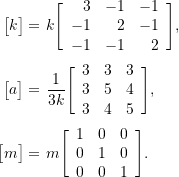### Approximate Methods for Multiple Degree of Freedom Systems: Chapter 9 Examples

### Example 9-1

At some instant in time, the masses in the system shown below are in positions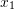and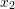and have velocities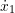and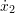respectively.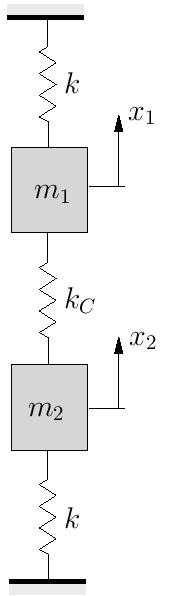1. Determine the potential and kinetic energies in the system in terms of,,andfrom first principles.
2. Show that the potential and kinetic energies can also be expressed as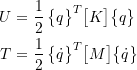where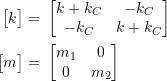and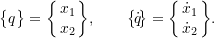### Example 9-2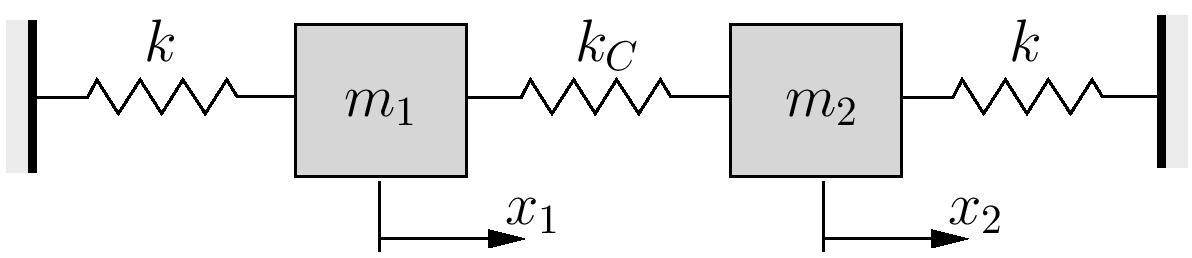1. For the system shown above, estimate the fundamental natural frequency using Rayleigh’s Quotient. Assume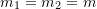and use trial vectors of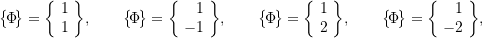and comment on the results.
It was previously determined that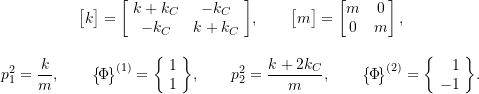2. Repeat the calculations in part (1) assuming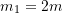,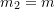, and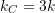.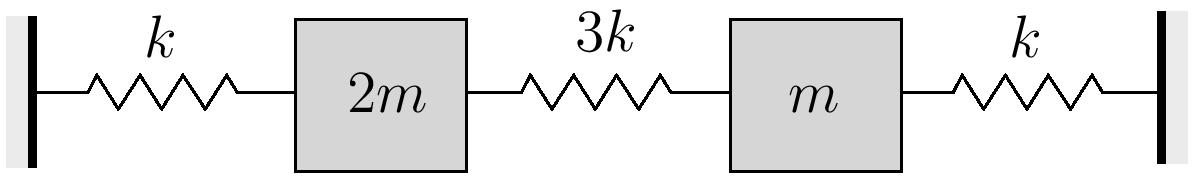Note that the exact solution in this case is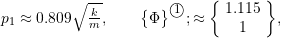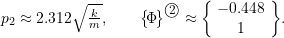### Example 9-3

Use Rayleigh’s method to estimate the fundamental natural frequency for a light simply supported beam supporting three equally spaced identical masses.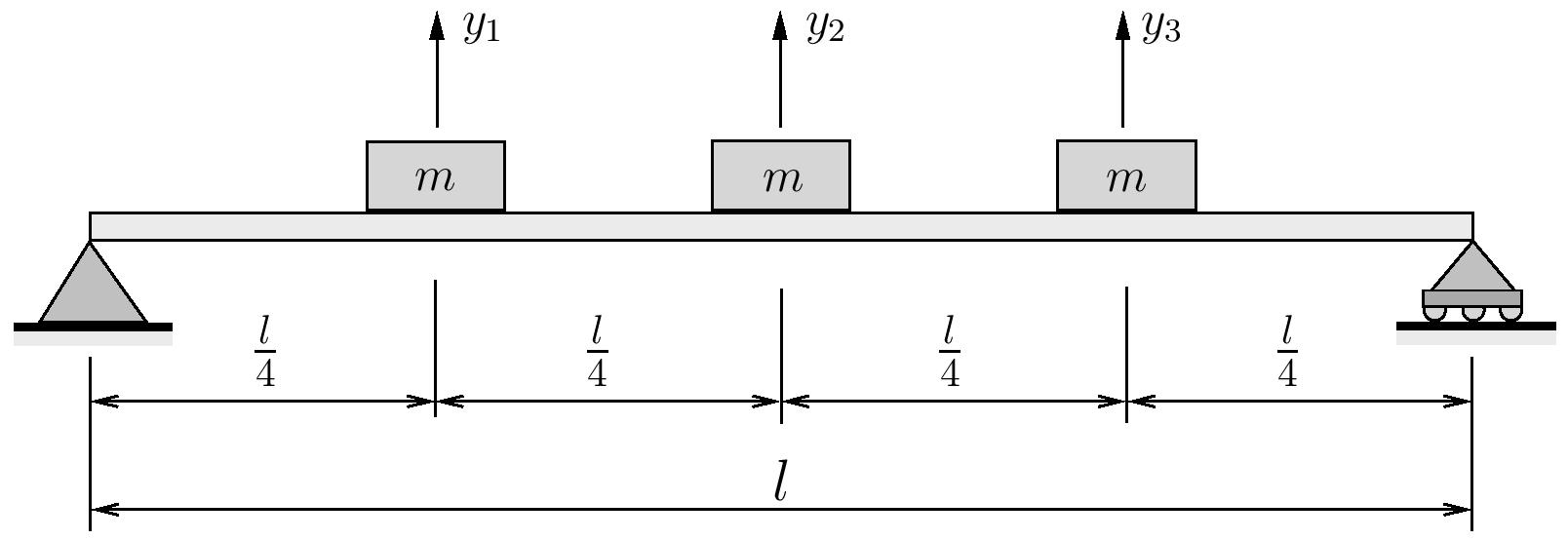Assume trial vectors of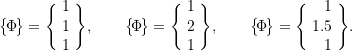Note that for this problem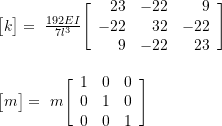### Example 9-4

Estimate the fundamental natural frequency for the system shown below using Dunkerley’s Method.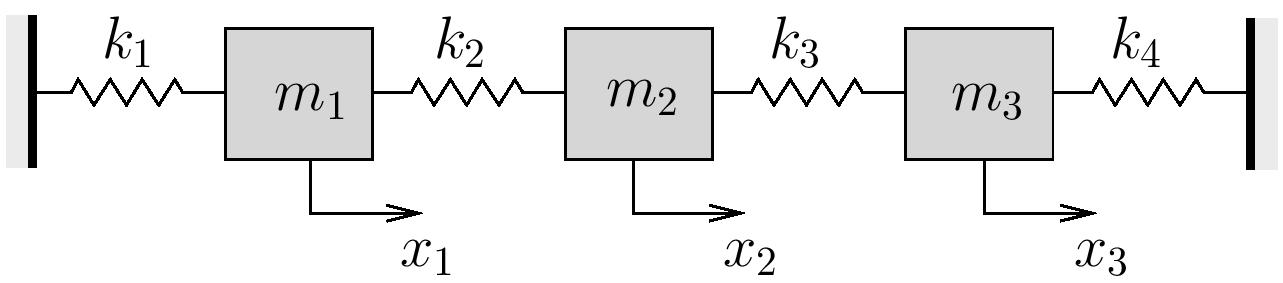Consider the following cases:

i)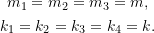ii)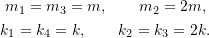iii)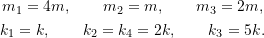Note that the stiffness matrix for this problem is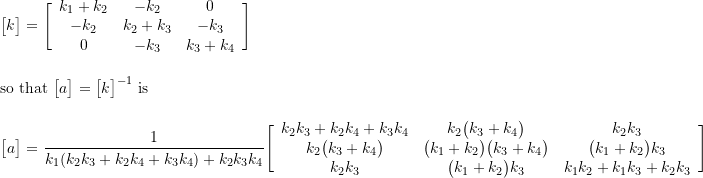### Example 9-5

Use Dunkerley’s method to estimate the fundamental natural frequency for a light simply supported beam supporting three equally spaced identical masses.Note that for this system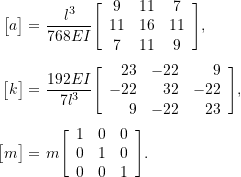### Example 9-6

For the system shown below: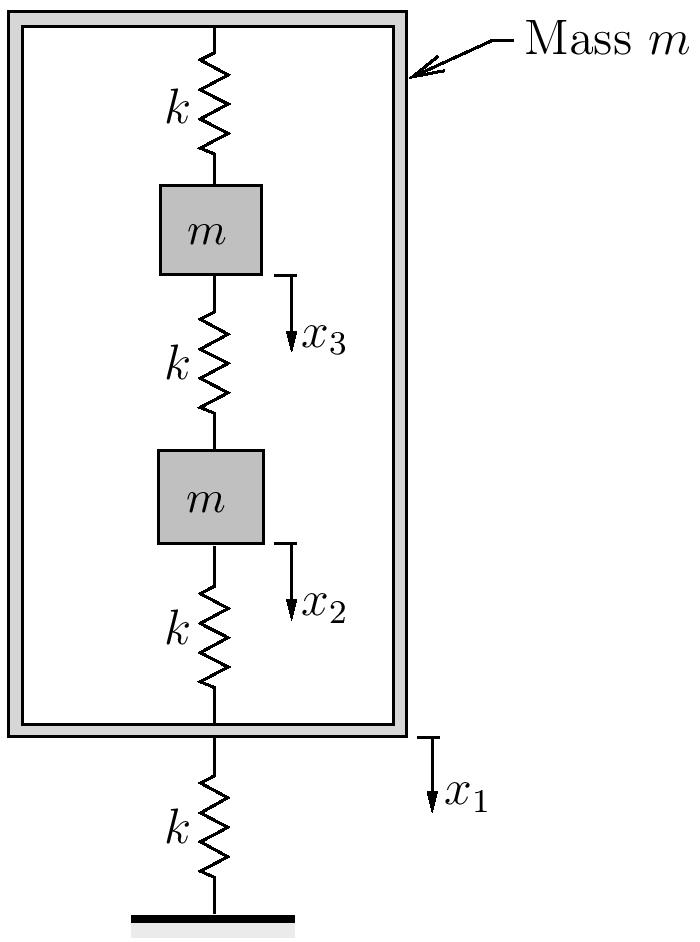1. Use matrix iteration to find the lowest and highest natural frequencies and associated mode shapes.
2. Use Rayleigh’s quotient to find an upper bound to the lowest natural frequency. Use a trial vector of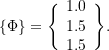3. Use Dunkerley’s method to find a lower bound for the lowest natural frequency.
4. Use Dunkerley’s method to find an upper bound for the highest natural frequency.

Note that for this problem,